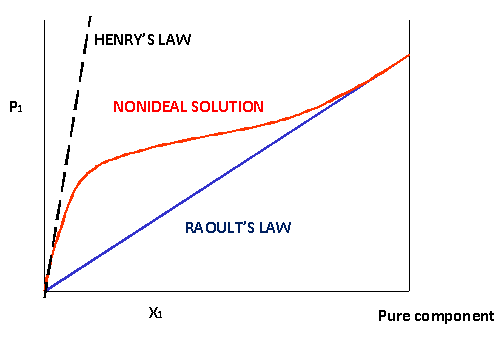# Henry's Law

$$\newcommand{\vecs}{\overset { \rightharpoonup} {\mathbf{#1}} }$$ $$\newcommand{\vecd}{\overset{-\!-\!\rightharpoonup}{\vphantom{a}\smash {#1}}}$$$$\newcommand{\id}{\mathrm{id}}$$ $$\newcommand{\Span}{\mathrm{span}}$$ $$\newcommand{\kernel}{\mathrm{null}\,}$$ $$\newcommand{\range}{\mathrm{range}\,}$$ $$\newcommand{\RealPart}{\mathrm{Re}}$$ $$\newcommand{\ImaginaryPart}{\mathrm{Im}}$$ $$\newcommand{\Argument}{\mathrm{Arg}}$$ $$\newcommand{\norm}{\| #1 \|}$$ $$\newcommand{\inner}{\langle #1, #2 \rangle}$$ $$\newcommand{\Span}{\mathrm{span}}$$ $$\newcommand{\id}{\mathrm{id}}$$ $$\newcommand{\Span}{\mathrm{span}}$$ $$\newcommand{\kernel}{\mathrm{null}\,}$$ $$\newcommand{\range}{\mathrm{range}\,}$$ $$\newcommand{\RealPart}{\mathrm{Re}}$$ $$\newcommand{\ImaginaryPart}{\mathrm{Im}}$$ $$\newcommand{\Argument}{\mathrm{Arg}}$$ $$\newcommand{\norm}{\| #1 \|}$$ $$\newcommand{\inner}{\langle #1, #2 \rangle}$$ $$\newcommand{\Span}{\mathrm{span}}$$$$\newcommand{\AA}{\unicode[.8,0]{x212B}}$$

Henry's law is one of the gas laws formulated by William Henry in 1803 and states: "At a constant temperature, the amount of a given gas that dissolves in a given type and volume of liquid is directly proportional to the partial pressure of that gas in equilibrium with that liquid." An equivalent way of stating the law is that the solubility of a gas in a liquid is directly proportional to the partial pressure of the gas above the liquid:

$C =k P_{gas}$

where

• $$C$$ is the solubility of a gas at a fixed temperature in a particular solvent (in units of M or mL gas/L)
• $$k$$ is Henry's law constant (often in units of M/atm)
• $$P_{gas}$$ is the partial pressure of the gas (often in units of atm)Figure $$\PageIndex{1}$$: Positive non-ideal behavior of the vapor pressure of a solution follows Henry's Law at low concentrations and Raoult's Law at high concentrations (pure).
##### Key differences between Raoult’s Law and Henry’s Law

Raoult's and Henry's laws are limiting laws, generally applicable when the solute concentration goes to zero. In this limit the vapor pressure of any component in the solution depends linearly on its mole fraction, implying the absence of solute-solute interactions.

Raoult's law describes the dependence of the vapor pressure of a solvent as a function of its mole fraction $$\chi_1$$:

$\lim_{\chi_1\rightarrow1}\left( \frac{p}{\chi_1}\right) =p^\ast \nonumber$

where $$p^{\ast}$$ is the vapor pressure of the pure solvent.

Henry's law describes the dependence of the vapor pressure of a solute as a function of its concentration. In terms of mole fraction $$\chi_2$$:

$$$\lim_{\chi_2\rightarrow0}\left( \frac{p}{\chi_2}\right) =K \nonumber$$$

For a binary mixture of pure substances it can be shown that the laws are complementary: if one law holds for one component then the other law holds for the other component. It can also be shown that the laws imply that the solution satisfies other criteria for ideality, including zero enthalpy and volume of mixing.

##### Example $$\PageIndex{1}$$

What is Henry's constant for neon dissolved in water given: $$C_{Ne}=23.5 \,mL/L$$ solution and STP (22,414 mL/mole gas) and pressure (1 atm)?

###### Solution

Now we can rearrange our equation from above to solve for the constant:

$C =k P_{Ne} \nonumber$

To use C we must convert 23.5 mL/L solution to Molarity. Since Ne is a gas, we can use our standard molar volume. Thus giving us:

$\dfrac{\text{23.5 mL/L soln}}{\text{1 mole Ne/22,414 mL}}= 0.00105\,M.$

Now we have solved for the solubility of Ne in the solution. C= 0.00105M and we know the pressure at STP is 1 atm, so we can now use our rearranged equation:

$k= \dfrac{C}{P_{Ne}} \nonumber$

Where C= 0.00105M, PNe = 1 atm, thus giving us k=0.00105 M/atm

##### Example $$\PageIndex{2}$$

Compute the molar solubility in water that is saturated with air.

###### Solution

This time, we need to use constant (k) that we just calculated and our $$P_{Ne}$$ in air.

\begin{align*} C &=k P_{gas} \\[4pt] &=(0.00105\; M/atm)(0.0341\; atm) \\[4pt] &= 3.58 \times 10^{-5}\; M \end{align*}

##### Applicability of Henry's Law
• Henry's law only works if the molecules are at equilibrium.
• Henry's law does not work for gases at high pressures (e.g., $$N_{2\;(g)}$$ at high pressure becomes very soluble and harmful when in the blood supply).
• Henry's law does not work if there is a chemical reaction between the solute and solvent (e.g., $$HCl_{(g)}$$ reacts with water by a dissociation reaction to generate $$H_3O^+$$ and $$Cl^-$$ ions).

## References

1. Zumdahl, Steven and Susan. Chemistry. Fifth Edition. Boston, MA: Houghton Mifflin Company, 2000.
2. Petrucci, Ralph, and William Harwood. F. Geoffrey Herring. Jeffry Madura. General Chemistry: Principles and Modern Applications. 9th ed. Upper Saddle River, NJ: Pearson, 2007.